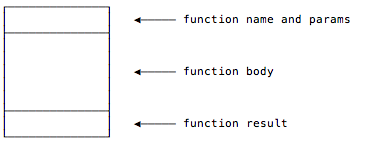recursion

This is a page from my book, Functional Programming, Simplified

Appendix: Recursion is Great, But ... (Scala’s fold and reduce)

“Folds can be used to implement any function where you traverse a list once, element by element, and then return something based on that. Whenever you want to traverse a list to return something, chances are you want a fold. That’s why folds are, along with maps and filters, one of the most useful types of functions in functional programming.”

From the book, Learn You a Haskell for Great Good!

This is a page from my book, Functional Programming, Simplified

Scala Recursion Lessons (Section)

This section contains lessons on recursion (recursive programming) in Scala. The lessons in this section are listed below.

This is a page from my book, Functional Programming, Simplified

Tail-Recursive Algorithms in Scala

“Tail recursion is its own reward.”

From the “Functional” cartoon on xkcd.com.

Goals

The main goal of this lesson is to solve the problem shown in the previous lessons: Simple recursion creates a series of stack frames, and for algorithms that require deep levels of recursion, this creates a `StackOverflowError` (and crashes your program).

This is a page from my book, Functional Programming, Simplified

A Visual Look at JVM Stacks and Frames

Given the background information of the previous lesson, let’s take a visual look at how the JVM stack and stack frames work by going back to our recursive sum function from the previous lesson.

Before the `sum` function is initially called, the only thing on the call stack is the application’s `main` method:Then main calls `sum` with `List(1,2,3)`, which I show here without the “List” to keep things simple:

This is a page from my book, Functional Programming, Simplified

JVM Stacks and Stack Frames

For functions without deep levels of recursion, there’s nothing wrong with the algorithms shown in the previous lessons. I use this simple, basic form of recursion when I know that I’m working with limited data sets. But in applications where you don’t know how much data you might be processing, it’s important that your recursive algorithms are tail-recursive, otherwise you’ll get a nasty `StackOverflowError`.

For instance, if you run the `sum` function from the previous lessons with a larger list, like this:

This is a page from my book, Functional Programming, Simplified

Recursion: Thinking Recursively

“To understand recursion,
one must first understand recursion.”

Stephen Hawking

Goal

This lesson has one primary goal: to show that the thought process followed in writing the `sum` function follows a common recursive programming “pattern.” Indeed, when you write recursive functions you’ll generally follow the three-step process shown in this lesson.

This is a page from my book, Functional Programming, Simplified

Recursion: A Conversation Between Two Developers

As an homage to one of my favorite Lisp books — an early version of what is now The Little Schemer — this lesson shows a little question and answer interaction that you can imagine happening between two Scala programmers.

Given this `sum` function:

``````def sum(list: List[Int]): Int = list match {
case Nil => 0
case x :: xs => x + sum(xs)
}``````

I hope this “conversation” will help drive home some of the points about how recursion works:

This is a page from my book, Functional Programming, Simplified

Recursion: Visualizing the recursive `sum` Function

Another way to view recursion is with visual diagrams. To demonstrate this, I’ll use this rectangular symbol in this lesson to represent a function:The first step

Using that symbol and a list with only three elements, the first `sum` function call looks like this: